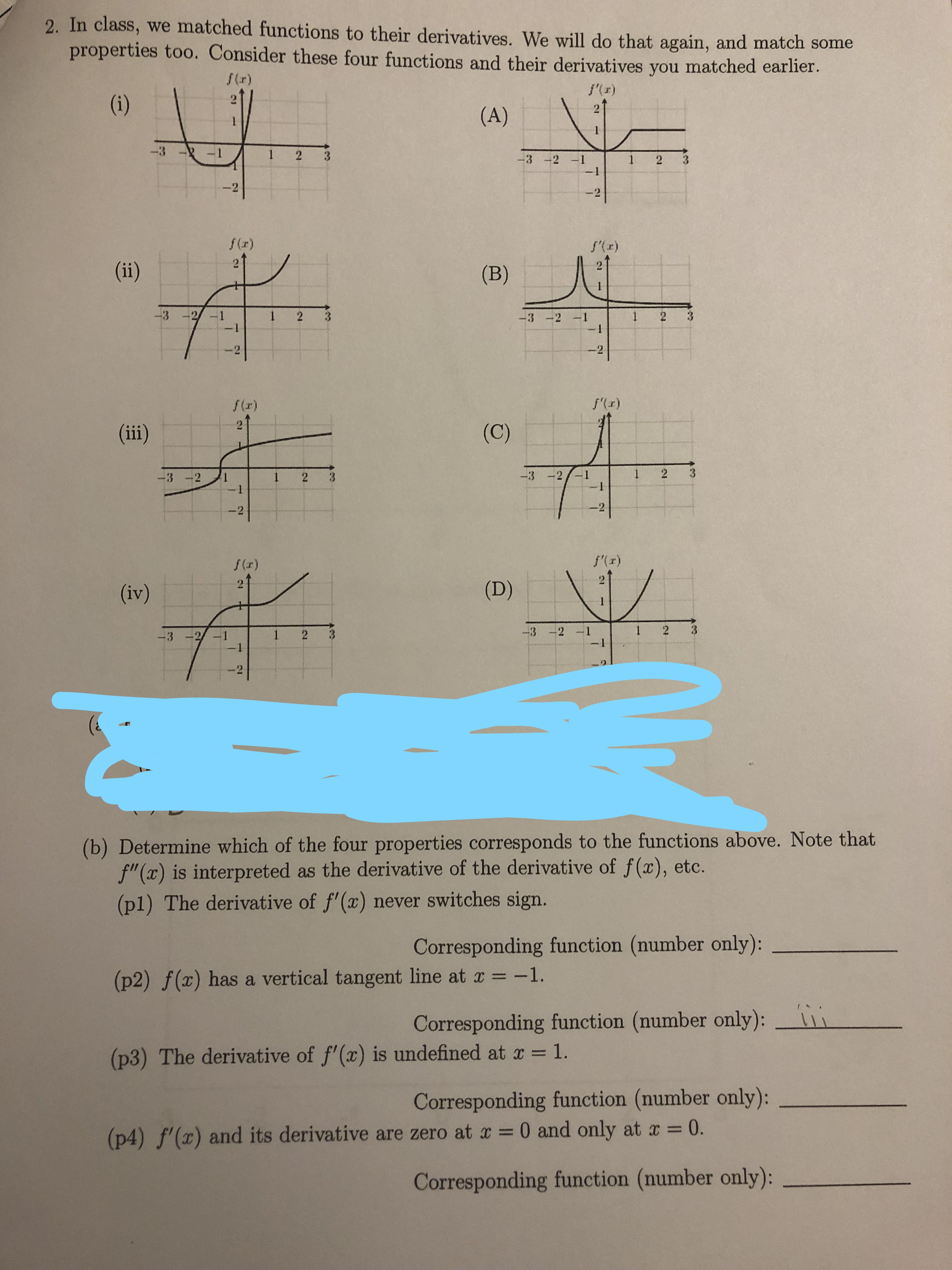# 2. In class, we matched functions to their derivatives. We will do that again, and match some properties too. Consider these four functions and their derivatives you matched earlier. f(r) f'() (i) 2 (A) -3 1 2 3 -3 -2 -1 -1 1 2 -2 -21 f(r) f(r) (ii) (B) 1 3 3 2 -1 2 1 2 -3 -2 -1 -1 -2 2 fr) f(x) (C) (ii) 2 -3: 3 -2 1 2 -2 2 f'(r) f(r) (D) (iv) 1 2 -3 -2 -1 -3 -2/ -1 1 2 1 (b) Determine which of the four properties corresponds to the functions above. Note that f"(x) is interpreted as the derivative of the derivative of f(x), etc. (p1) The derivative of f'(x) never switches sign. Corresponding function (number only): (p2) f(x) has a vertical tangent line at x -1. Corresponding function (number only): (p3) The derivative of f'(x) is undefined at x 1. Corresponding function (number only): 11 (p4) f'(x) and its derivative are zero at x 0 and only at x 0. 1 Corresponding function (number only): 3 3

Question

Just need Question 2b, p1-p4help_outlineImage Transcriptionclose2. In class, we matched functions to their derivatives. We will do that again, and match some properties too. Consider these four functions and their derivatives you matched earlier. f(r) f'() (i) 2 (A) -3 1 2 3 -3 -2 -1 -1 1 2 -2 -21 f(r) f(r) (ii) (B) 1 3 3 2 -1 2 1 2 -3 -2 -1 -1 -2 2 fr) f(x) (C) (ii) 2 -3: 3 -2 1 2 -2 2 f'(r) f(r) (D) (iv) 1 2 -3 -2 -1 -3 -2/ -1 1 2 1 (b) Determine which of the four properties corresponds to the functions above. Note that f"(x) is interpreted as the derivative of the derivative of f(x), etc. (p1) The derivative of f'(x) never switches sign. Corresponding function (number only): (p2) f(x) has a vertical tangent line at x -1. Corresponding function (number only): (p3) The derivative of f'(x) is undefined at x 1. Corresponding function (number only): 11 (p4) f'(x) and its derivative are zero at x 0 and only at x 0. 1 Corresponding function (number only): 3 3 fullscreen